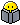Hi Folks,

In an official cisco cert. guide there is a review question about subnetting that I don't really understand. Answer a. seemed to me the best answer, followed by b., but the book says these are wrong. What are the two workable solutions and especially why?

3. A Class B network needs to be subnetted such that it supports 100 subnets and 100 hosts/
subnet. Which of the following answers list a workable combination for the number of
network, subnet, and host bits? (Select two answers.)
a. Network = 16, subnet = 7, host = 7
b. Network = 16, subnet = 8, host = 8
c. Network = 16, subnet = 9, host = 7
d. Network = 8, subnet = 7, host = 17

Richard

• B and C looked appropriate to me. A is wrong (since 16+7+7=30Ohh boy..!! where are the remaining 2 bits).B is right since 2^8=256 networks and (2^8 )-2= 254 hosts/each subnet. C is right since 2^9=512 networks and (2^7)-2=126 hosts/subnet.
2016 Certification Goals: CCNP Route /COLOR][B][/B][I][B]X[/B][/I][COLOR=#008000-->-->TShoot[], CCDP []
• Thanks Nans,

I see now, the address should alway be 32 bits and the fixed network part for a Class B address is 16 bits.

Great!
• its the question wording that makes it complete mess,where simple /22 /23 /24 /25 would of make it life easier -since youd only need to know values of the bits instead of looking at random numbers and adding them up and most likely making mistake

tricky part is the /25 that someone doing on a whim could select as one of the options-thus going way over top on subnets.
• Member Posts: 1,340 ■■■■□□□□□□
B and C since A doesn't have enough bits. Now, on the real thing they will probably ask you to either --

1) Give the answer that provides the most hosts/subnets, while allowing for at least 100 subnets.
2) Give the answer that provides the most subnets, while providing at least 100 hosts/subnet.

The best answer for 1 would be A, but with 9 Host Bits (128 Subnets of 510 Hosts). If that isn't an option, then it would be B (256 Subnets of 254 Hosts). For 2, C (512 Subnets of 126 Hosts) would be the best answer.

A good strategy is to eliminate the obvious wrong answers. In any MC question, 1 or 2 will typically be obviously wrong at first glance. In this case, D could be eliminated immediately as a Class B has 16 Network Bits.
R&S: CCENT CCNA CCNP CCIE [ ]
Security: CCNA [ ]
Virtualization: VCA-DCV [ ]